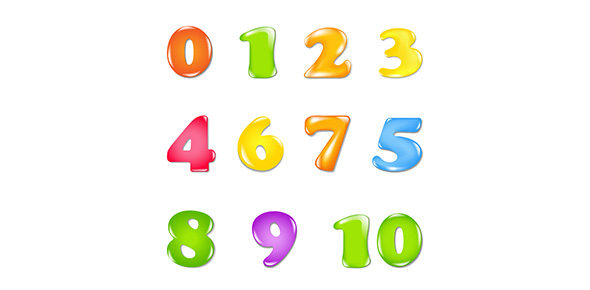# Number Systems

15 QuestionsSettingsRelated Topics
• 1.
In between two rational number there is/are:
• A.

Exactly one rational number

• B.

Infinitely many rational number

• C.

Many irrational numbers

• D.

Only irrational numbers

• 2.
The product of a rational and an irrational numbers is:
• A.

Always an integer

• B.

Always a rational number

• C.

Always an irrational number

• D.

Sometimes rational and sometimes irrational

• 3.
The decimal expansion of an irrational number may be:
• A.

Terminating

• B.

Recurring

• C.

Either terminating or non- terminating

• D.

Non-terminating and non-recurring

• 4.
A rational number betweenand:
• A.
• B.
• C.
• D.
• 5.
Which of the following is the irrational?
• A.
• B.
• C.
• D.
• 6.
is equal to:
• A.
• B.
• C.
• D.
• 7.
Which of the following is irrational?
• A.
• B.
• C.
• D.
• 8.
is equal to:
• A.
• B.
• C.
• D.
• 9.
Which of the following is equal to x2?
• A.
• B.
• C.
• D.
• 10.
The value of  is:
• A.
• B.
• C.
• D.
• 11.
equals:
• A.
• B.
• C.
• D.
• 12.
What would be the denominator after rationalizing?
• A.

19

• B.

20

• C.

25

• D.

35

• 13.
The product equals:
• A.
• B.
• C.
• D.
• 14.
If, then  is equal to:
• A.

2.732

• B.

0.2679

• C.

0.732

• D.

0.5358

• 15.
Value of  is:
• A.

4

• B.

16

• C.

64

• D.

256.25